# 二维带电粒子束的相空间分布和发射度

2016年 9月 19日

### 离子束和电子束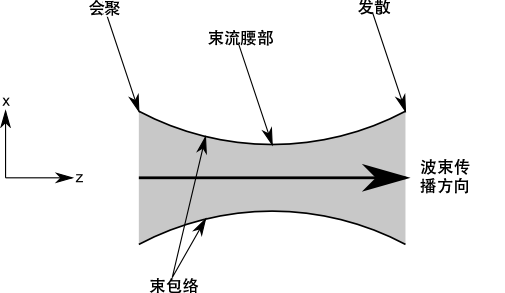### 比较层束流和非层束流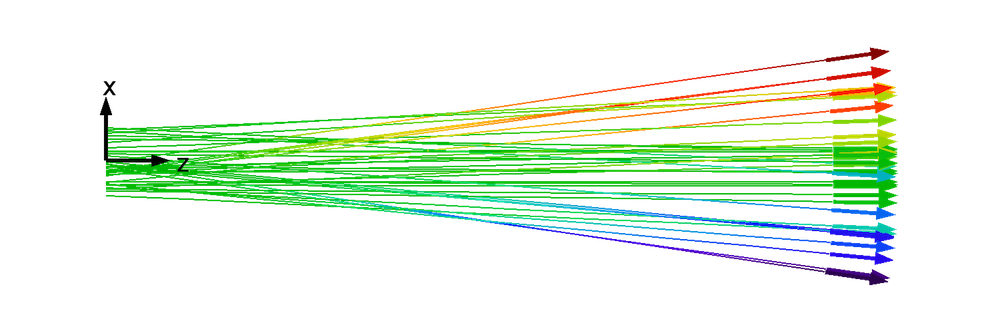1. 横向位置和速度之间存在一一对应的关系。在任意的横向位置上，电子束粒子的路径不会发生交叉。唯一的例外是会聚电子束，此类束流的全部粒子将交汇于同一点。
2. 横向位置和速度的比例关系是呈线性的。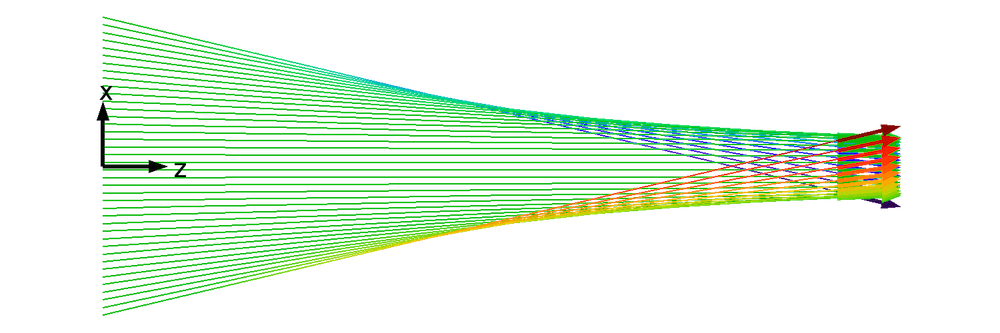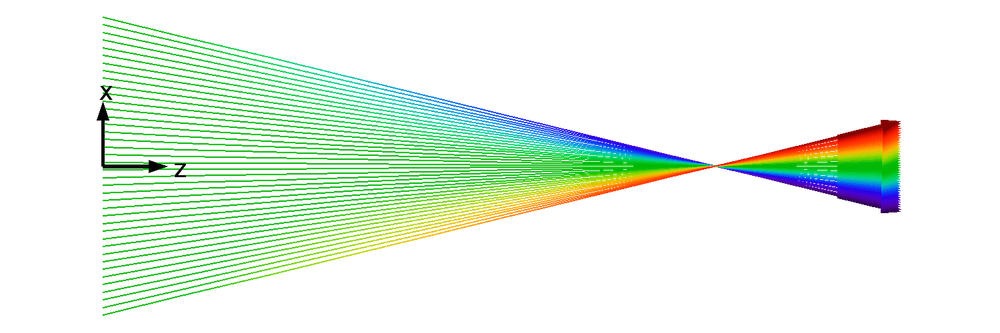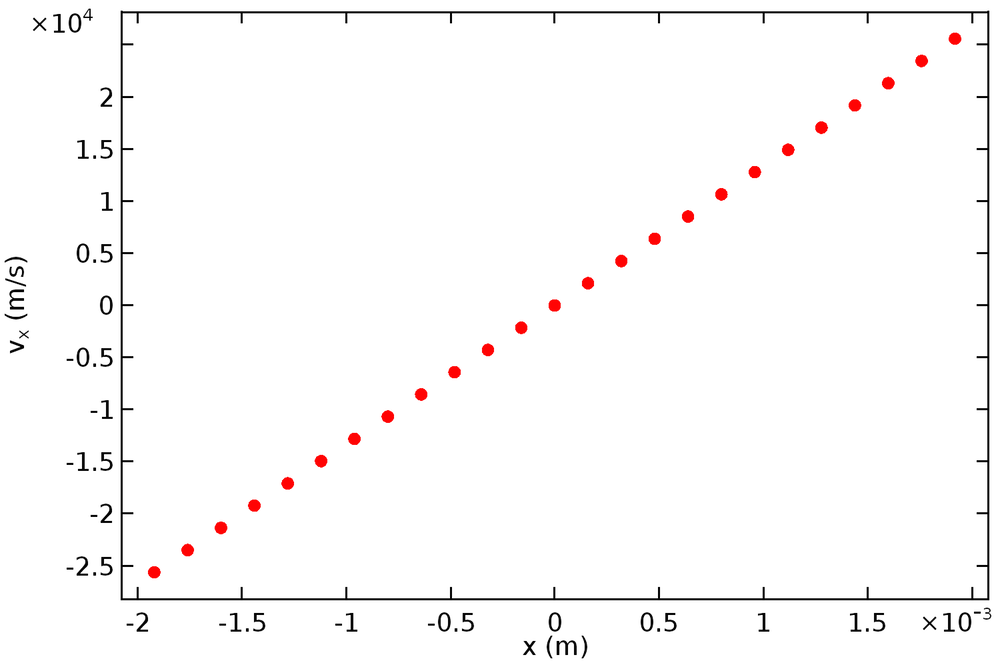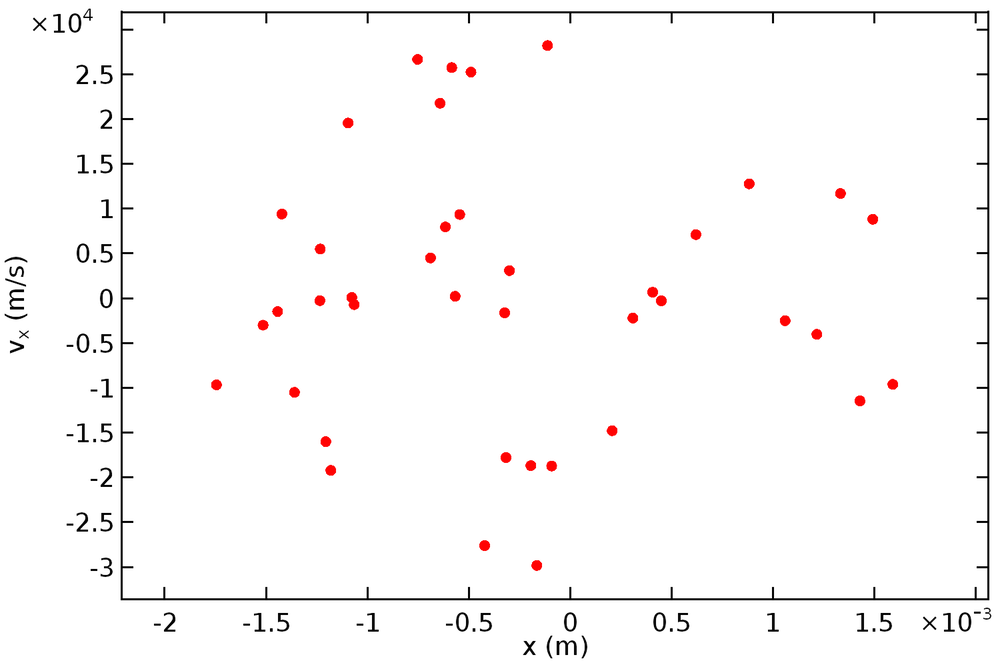### 束流发射度简介

\gamma x^2 + 2\alpha x x' + \beta x'^2 = \varepsilon

(1)

\gamma \beta -\alpha^2 = 1

• 相对于方程（1）使用的其他参数而言，γ 是最常用的的参数，它用于描述束流的比例。ε 恒定不变，γ 增加时，束流占据的空间区域将随之减小（x 值范围缩小），与此同时速度分布随之增大（x’ 值范围扩大）。
• α 用于描述迹空间椭圆的倾斜度。在直立椭圆中，α 对应的是束流腰部，且 α = 0。若 α > 0，则为会聚束流；若 α < 0，则为发散束流。
• β 也被称为振幅函数电子感应加速函数，用于描述束流的比例。ε 恒定不变，β 增加时，束流占据的空间区域将随之增大（x 值范围扩大），与此同时速度分布随之减小（x’ 值范围缩小）。
• ε 用于描述迹空间椭圆的大小，又被称为发射度。因为我们正在讨论横向位置和动量，所以将其称为横向发射度 会更加形象具体。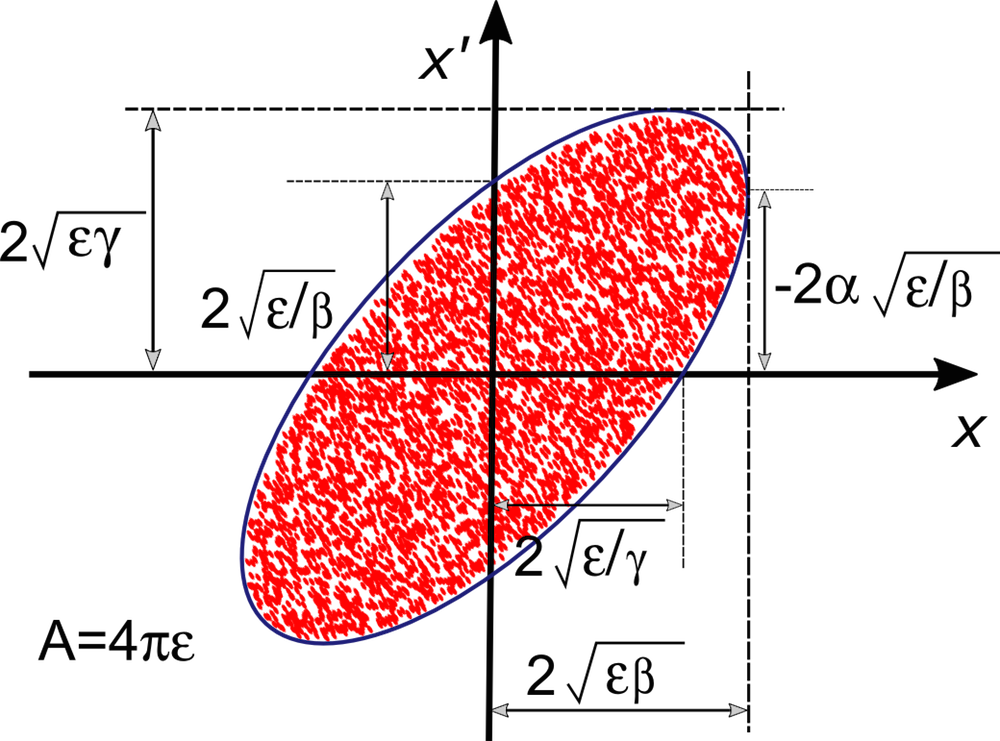### 从统计学角度解释发射度

(2)

\varepsilon = \sqrt{\left\textless\left{(x -(x))}^2\right>
\left\textless\left{(x' -(x'))}^2\right>
– \left\textless\left{(x- (x))\left(x' -(x'))\right>^2}

(x) = \frac{1}{N}\sum_{i=1}^{N} x_i

(3)

\varepsilon = 4\sqrt{\left\textless\left(x -(x))^2\right>
\left\textless\left(x' -(x'))^2\right>
– \left\textless\left(x- (x))\left(x'-(x&#39)\right)\right>^2}

\varepsilon_{1,\textrm{rms}} = \sqrt{(x^2)(x'^2) -(xx')^2}

\begin{aligned}
\gamma &= \frac{(x'^2)}{\varepsilon_{1,\textrm{rms}}} \\
\beta &= \frac{(x^2)}{\varepsilon_{1,\textrm{rms}}} \\
\alpha &= -\,\frac{(xx')}{\varepsilon_{1,\textrm{rms}}}
\end{aligned}

### 有关束流发射度的讨论

• 束流尺寸较小（x 值范围减小）
• 速度分布较窄（x’ 值范围减小）

### 参考文献

1. Humphries, Stanley. Charged Particle Beams. Courier Corporation, 2013.
2. Davidson, Ronald C., and Hong Qin. Physics of intense charged particle beams in high energy accelerators. Imperial college press, 2001.